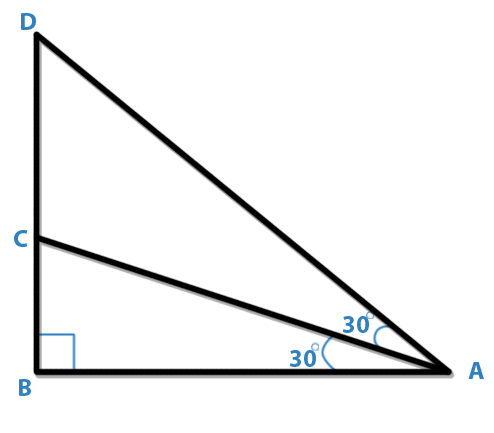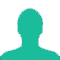# In the following figure which of the following statements is true?A. AB = BD

B. AC = CD

C. BC + CD

### Solution(By Examveda Team)

In Triangle ABD,
∠BAD + ∠B + 90° = 180°
or, ∠BAD + ∠B = 90° - - - - - - - (1)

Now, in Triangle ABC,
∠ACB + ∠B + ∠A = 180°
∠ACB + ∠B = 180° - 90°
∠ACB = 90° - ∠B - - - - - (2)
From (1) and (2), ∠BAD = ∠ACB
So, AC = CD

This Question Belongs to Arithmetic Ability >> Triangles

1.In equation 1 --- angle BAD + angle B = 90
cannot be true unless angle BAD = 0
since angle B itself is 90

2.Can't agree..

3.can't agree

4.i agreed it well explaination

5.In the figure angle B is 90° then how can u say that BAD+B+90=180

6.good solution explained by Sannyasi. but the explanation provided by examveda is complete crap..

7.In Triangle ABD,
∠B=90,∠A=30+30=60degre
then ∠D=30degre
now Consider Triangle ACD
∠D=30,∠DAC=30
These two side is Opposite of two equal angle So, AC = CD.

8.Not agree could u explain further

9.I don't understand. Can explain more clearly please? Thank you

10.i dont agree

Related Questions on Triangles